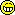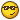Has this serial number been tampered with?

Recommended Posts

Guys,

This is a 2003 DRZ400E,

Does this stamping look suspiscious? The "4200" is at a slight angle and the gap between the last "0" of the "4200" and the following "1" looks too big.  Has this been tampered with?

Edited by segge

Share on other sites

Guys,

This is a 2003 DRZ400E,

Does this stamping look suspiscious? The "4200" is at a slight angle and the gap between the last "0" of the "4200" and the following "1" looks too big.  Has this been tampered with?

Not to me....all the numbers are made with the same stamp, font, etc...

Share on other sites

So, you are asking for a critique of your work?(couldn't resist the comment, I have no opinion on the actual question)

•2

Share on other sites

It looks legit but... does it match the title or MSO?

Often, serial numbers  have been imprinted using different typeface fonts to make re-stamping more difficult to match, E, a larger set of stamps must be owned

Share on other sites

I'm in Senegal, Bought the bike from a guy who left the country. I took the bike in for inspection and they say there is a problem with the number

Edited by segge
•1

Share on other sites

Looks well dodgy to me - its not straight. Very amateurish attempt

•2

Share on other sites

under the 300 are those grinder marks

Share on other sites

Looks suspect for sure,  the 2 0's in the middle are lower then the rest,  you can kinda see marks on the metal,  i would not buy that bike.

•1

Share on other sites

the numbers should be in a straight line

Share on other sites

First digit - Country code
Japan (J)

Second digit - Manufacturer

Suzuki (S)

Third digit - Identifier

Motorcycle (1 or A)

Fourth digit - Vehicle category

Business model or commuter (WRONG

Fifth digit - Engine Displacement

Data here is Suzuki specific, haven't found info for other brands.
A=49cc and less
B=50-69cc
C=70-79cc
D=80-89cc
E=90-99cc
F=100-124cc
G=125-149cc
H=150-199cc
J=200-249cc
K=250-399cc WRONG SHOULD BE A LETTER NOT NUMBER IN THIS POSITION

Sixth digit - Engine Type
1=2 stroke single WRONG SHOULD BE A NUMBER 4 IN THIS POSITION

Seventh digit
Data here is Suzuki specific, haven't found info for other brands.
Design sequence or model version first version uses 1 then second version uses 2 etc... the tenth version would then be identified by the letter A then B etc..
Eigth digit
Data here is Suzuki specific, haven't found info for other brands.
First version uses 1 then second version uses 2 etc... the tenth version would then be identified by the letter A then B etc...Ninth digit - Check digit
The ninth vehicle identification number digit is a VIN accuracy check digit, verifying the previous VIN numbers. This is how the check digit works

STEP 1
Assign to each number in the VIN its actual mathematical value and assign to each letter the value specified for it in the following chart:
ASSIGNED VALUES:
A=1 G=7 P=7 X=7
B=2 H=8 R=9 Y=8
C=3 J=1 S=2 Z=9
D=4 K=2 T=3
E=5 M=3 U=4
F=6 N=4 V=5

STEP 2
Multiply the assigned value for each character in the VIN by the position weight factor specified in the following chart:
VIN POSITION AND WEIGHT FACTOR:
1st = 8 10th = 9
2nd = 7 11th = 8
3rd = 6 12th = 7
4th = 5 13th = 6
5th = 4 14th = 5
6th = 3 15th = 4
7th = 2 16th = 3
8th = 10 17th = 2
9th = check digit

STEP 3
Add the products from steps 1 and 2 and divide the total by 11.

STEP 4
The numerical remainder is the check digit which would appear in the 9th position in the VIN. If the remainder is 10, then the letter "X" is used for the check digit.
WRONG cHECK NUMBER

•6

Share on other sites
•2

Share on other sites

The one digit is crooked. Looks suspicious to me

Share on other sitesVIN.jpg

First digit - Country code

Japan (J)

Second digit - Manufacturer

Suzuki (S)

Third digit - Identifier

Motorcycle (1 or A)

Fourth digit - Vehicle category

Business model or commuter (WRONG

Fifth digit - Engine Displacement

Data here is Suzuki specific, haven't found info for other brands.

A=49cc and less

B=50-69cc

C=70-79cc

D=80-89cc

E=90-99cc

F=100-124cc

G=125-149cc

H=150-199cc

J=200-249cc

K=250-399cc WRONG SHOULD BE A LETTER NOT NUMBER IN THIS POSITION

Sixth digit - Engine Type

1=2 stroke single WRONG SHOULD BE A NUMBER 4 IN THIS POSITION

Seventh digit

Data here is Suzuki specific, haven't found info for other brands.

Design sequence or model version first version uses 1 then second version uses 2 etc... the tenth version would then be identified by the letter A then B etc..

Eigth digit

Data here is Suzuki specific, haven't found info for other brands.

First version uses 1 then second version uses 2 etc... the tenth version would then be identified by the letter A then B etc...Ninth digit - Check digit

The ninth vehicle identification number digit is a VIN accuracy check digit, verifying the previous VIN numbers. This is how the check digit works

STEP 1

Assign to each number in the VIN its actual mathematical value and assign to each letter the value specified for it in the following chart:

ASSIGNED VALUES:

A=1 G=7 P=7 X=7

B=2 H=8 R=9 Y=8

C=3 J=1 S=2 Z=9

D=4 K=2 T=3

E=5 M=3 U=4

F=6 N=4 V=5

STEP 2

Multiply the assigned value for each character in the VIN by the position weight factor specified in the following chart:

VIN POSITION AND WEIGHT FACTOR:

1st = 8 10th = 9

2nd = 7 11th = 8

3rd = 6 12th = 7

4th = 5 13th = 6

5th = 4 14th = 5

6th = 3 15th = 4

7th = 2 16th = 3

8th = 10 17th = 2

9th = check digit

STEP 3

Add the products from steps 1 and 2 and divide the total by 11.

STEP 4

The numerical remainder is the check digit which would appear in the 9th position in the VIN. If the remainder is 10, then the letter "X" is used for the check digit.

WRONG cHECK NUMBER

Is this in FACTS? VERY USEFUL.

•1

Share on other sites

All that above said..

Republic of Senegal, a country in West Africa may have it's own procedures for marking (changing) a VIN after a theft recovery, importation, insurance total, custom build? ect.

​I have no personal experience or expert knowledge of how Senegal handles such things.

Create an account or sign in to comment

You need to be a member in order to leave a comment